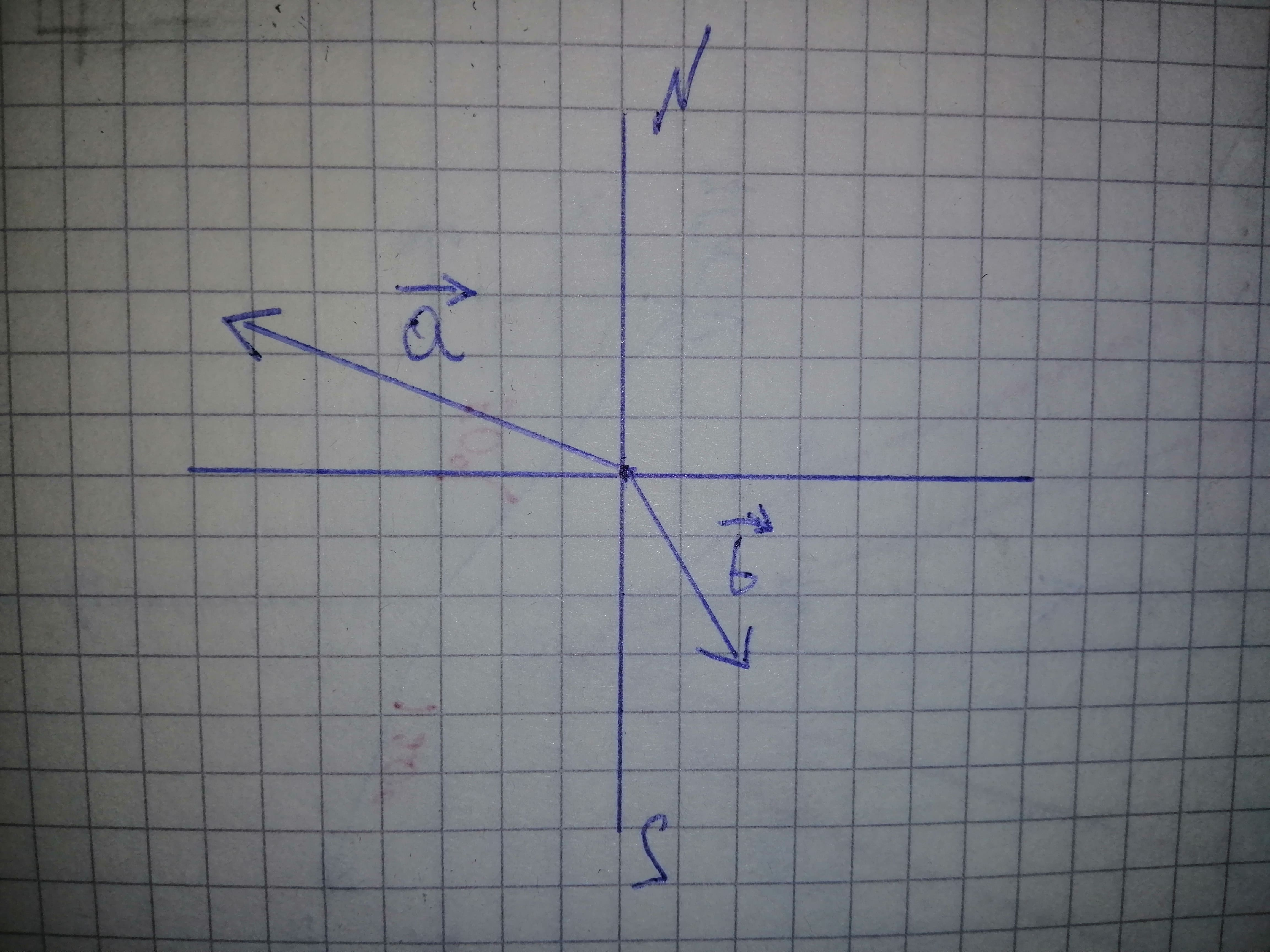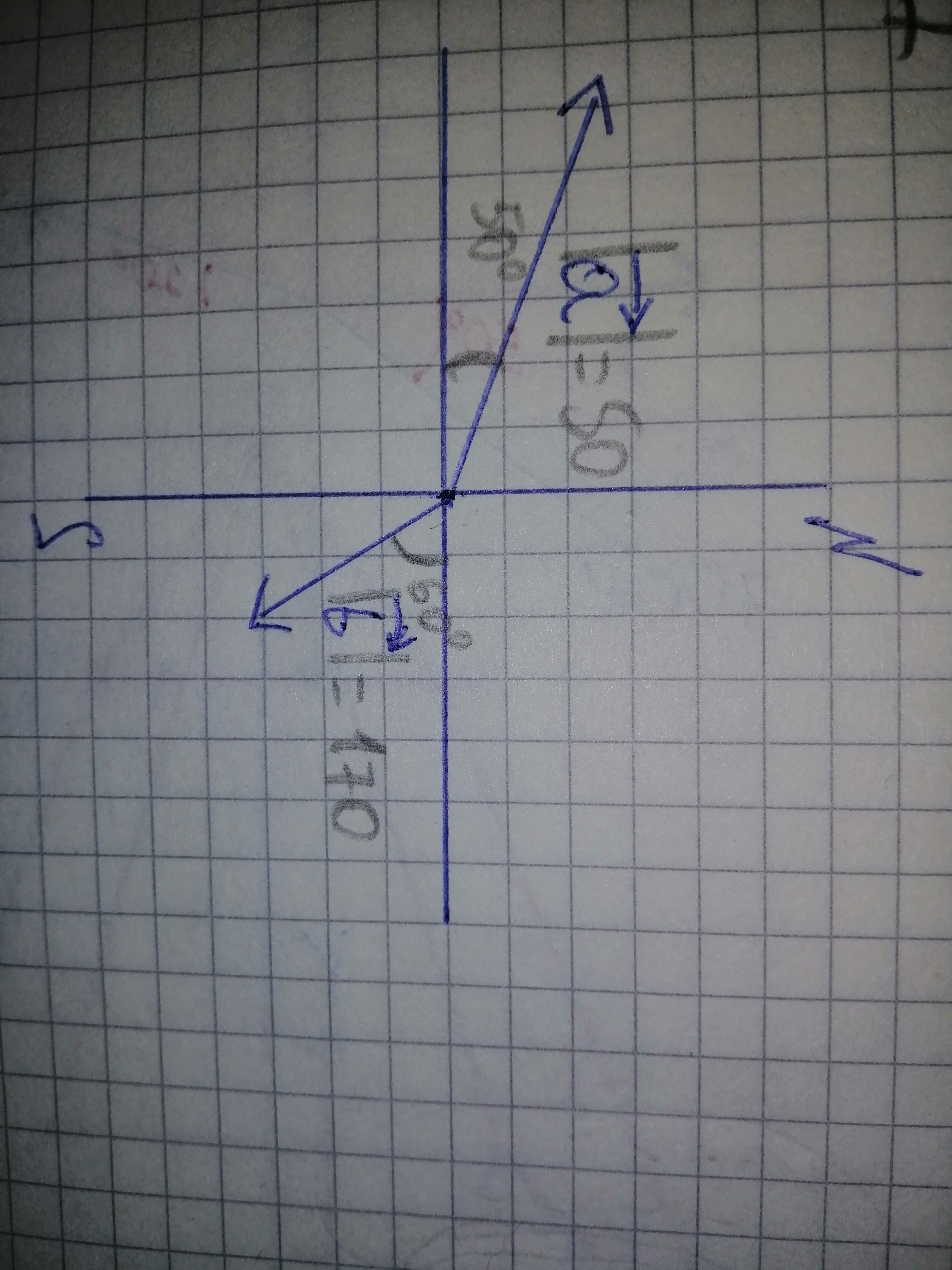iohanetc

2020-10-25

Find the sum of the given vectors
$50N\mathrm{\angle }{320}^{\circ }+170N\mathrm{\angle }{150}^{\circ }$
$Rx=?$
$Ry=?$
$R=?$
$\theta =?$Luvottoq

Step 1
Assume that the vectors are A and B. Draw the vectors as symbols on the graph.
$\stackrel{\to }{a}=50N\mathrm{\angle }{320}^{\circ }$
$\stackrel{\to }{b}=170N\mathrm{\angle }{150}^{\circ }$Step 2
Now, resolve the vectors a and b to get$|{\stackrel{\to }{a}}_{x}|=-50\mathrm{cos}{50}^{\circ }$
$|{\stackrel{\to }{a}}_{y}|=50\mathrm{sin}{50}^{\circ }$
$|{\stackrel{\to }{b}}_{x}|=170\mathrm{cos}{60}^{\circ }$
$|{\stackrel{\to }{b}}_{y}|=-170\mathrm{sin}{60}^{\circ }$
${R}_{x}=|{\stackrel{\to }{a}}_{x}|+|{\stackrel{\to }{b}}_{x}|$
${R}_{x}=-50\mathrm{cos}{50}^{\circ }+170\mathrm{cos}{60}^{\circ }$
${R}_{x}=52.86$
${R}_{y}=|{\stackrel{\to }{a}}_{y}|+|{\stackrel{\to }{b}}_{y}|$
${R}_{y}=50\mathrm{sin}{50}^{\circ }-170\mathrm{sin}{60}^{\circ }$
${R}_{y}=-108.92$
Step 3
Finally, determine the resultant vector R and the direction theta using the resultant vectors
$R=\sqrt{{R}_{x}^{2}+{R}_{y}^{2}}$
$=\sqrt{{\left(52.86\right)}^{2}+{\left(-108.92\right)}^{2}}$
$=\sqrt{14657.746}$
$=121.07$
Hence, $R=121.07$
$\theta ={\mathrm{tan}}^{-1}\left(\frac{{R}_{y}}{{R}_{x}}\right)$
$={\mathrm{tan}}^{-1}\left(\frac{-180.95}{52.86}\right)$
$=-{64.11}^{\circ }$
$={295.89}^{\circ }$
$\approx {296}^{\circ }$
Hence, theta $={296}^{\circ }$ from horizontal axis.

Do you have a similar question?